Finding point when tangent is parallel/ perpendicular

Chapter 6 Class 12 Application of Derivatives
Concept wise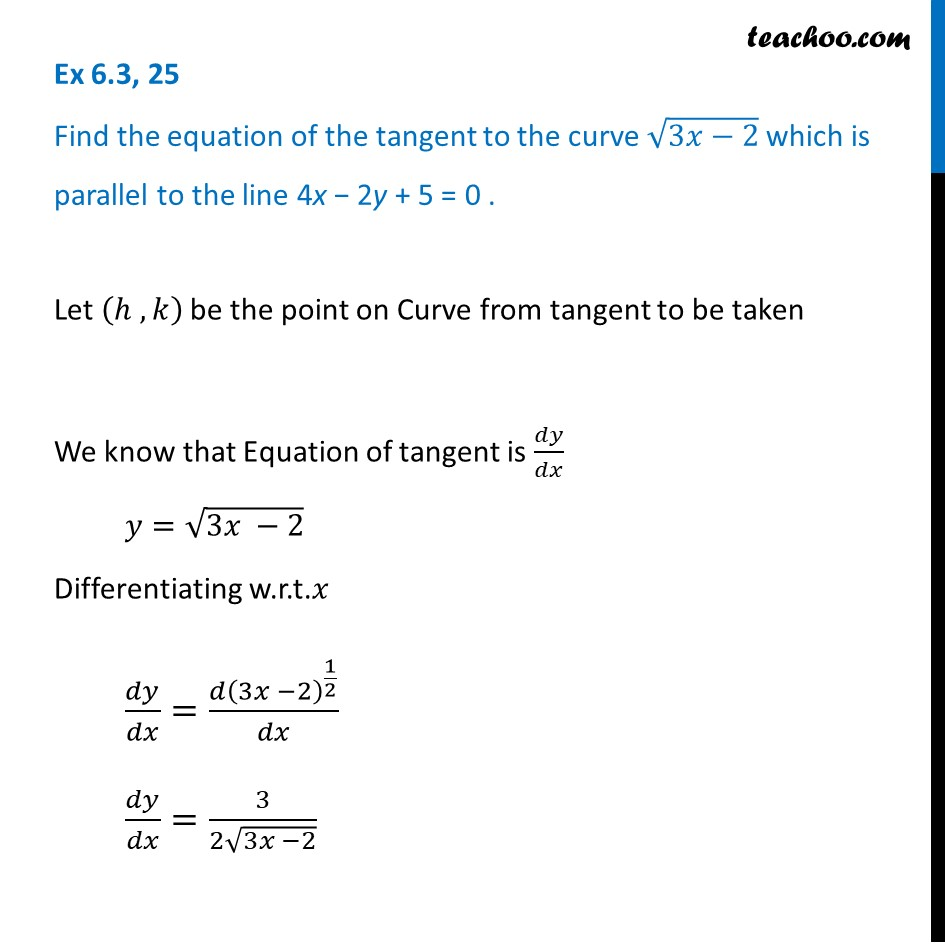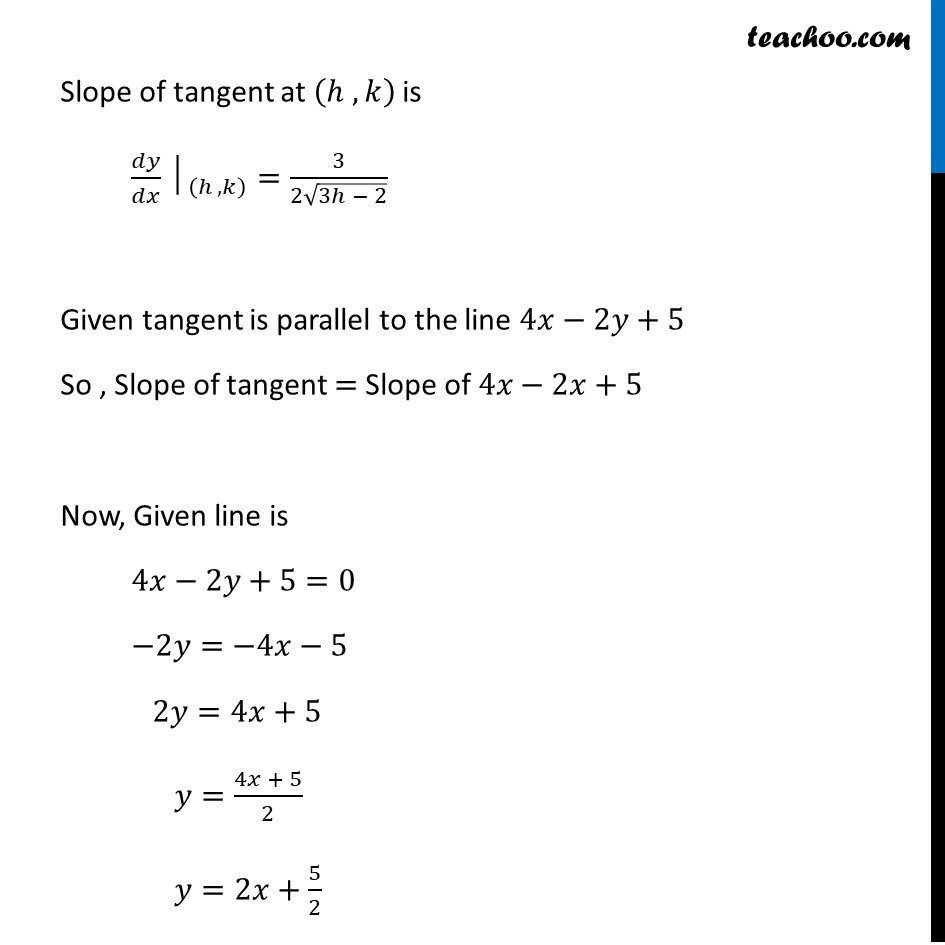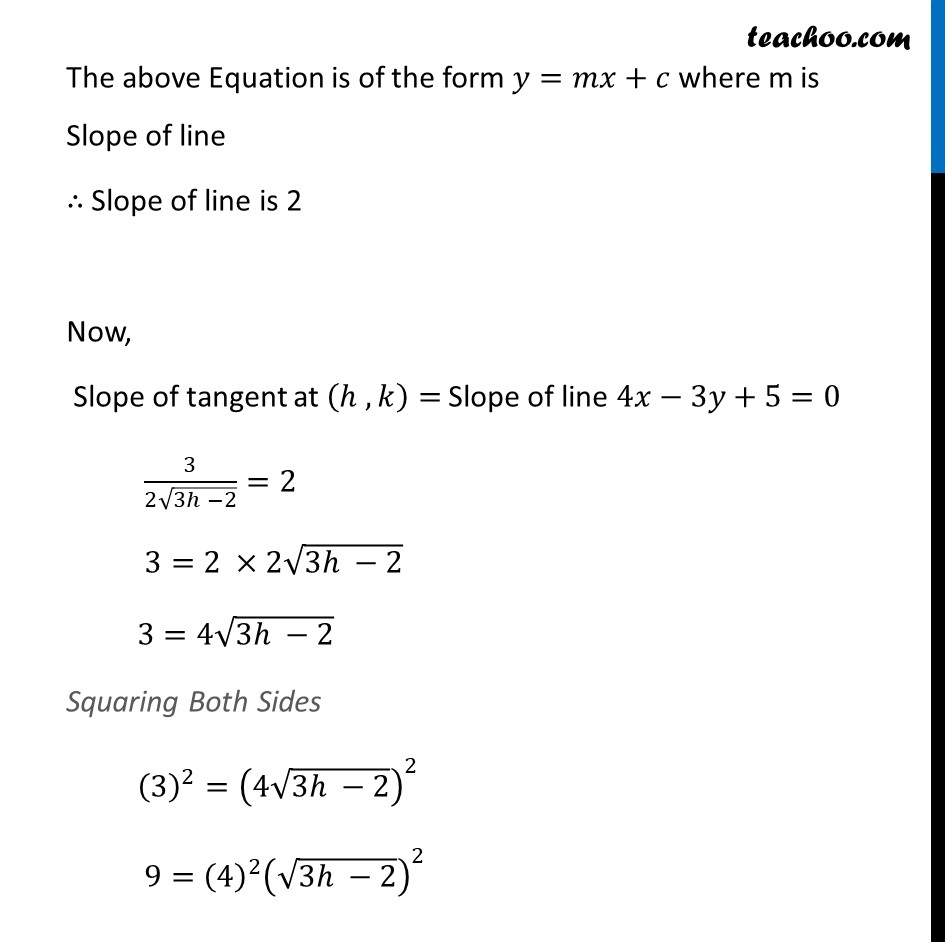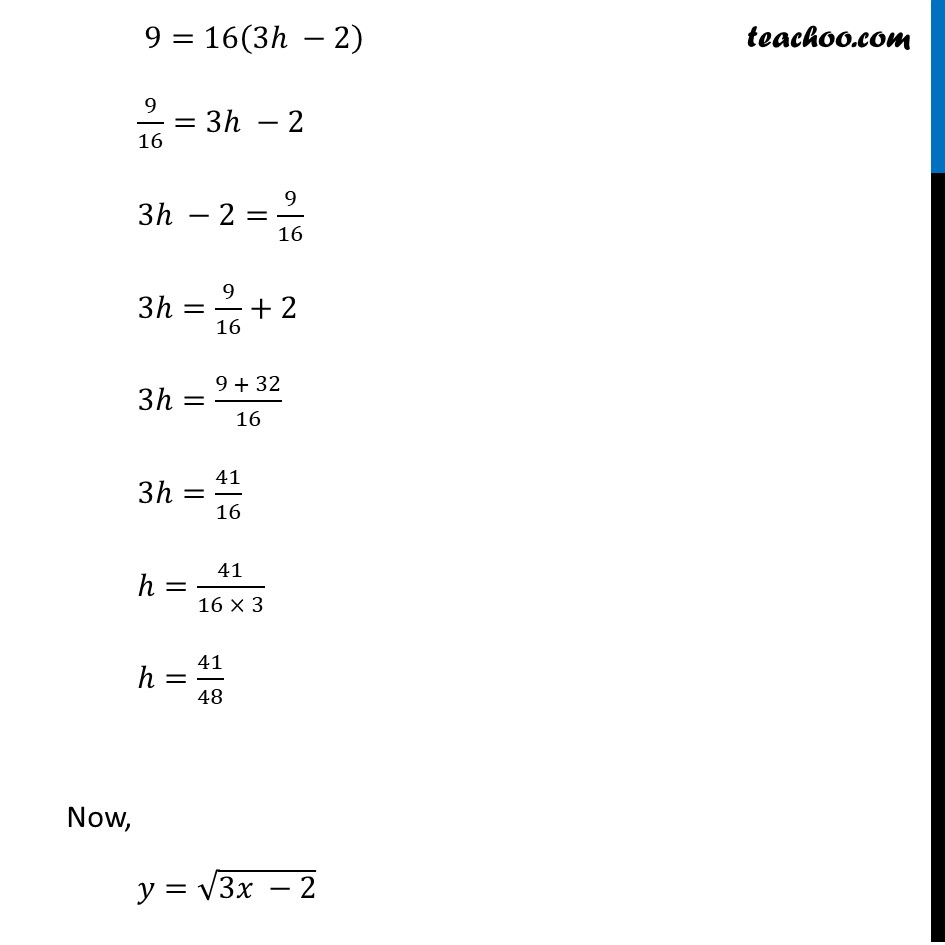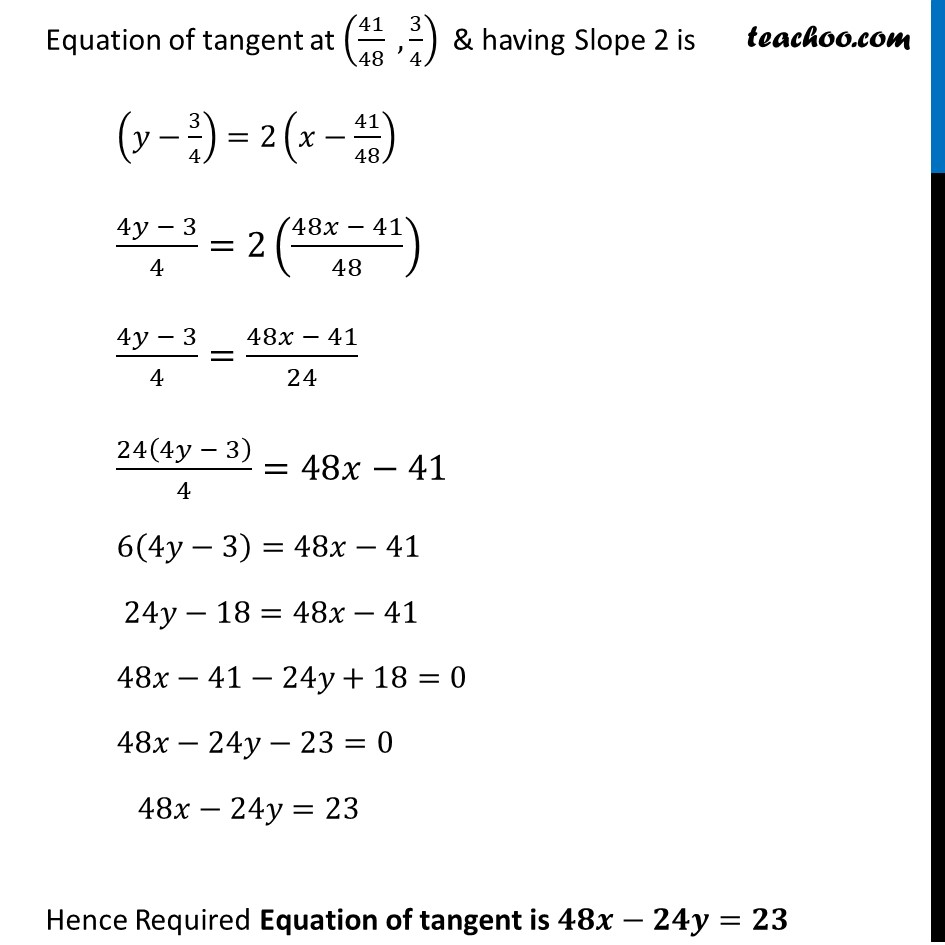Introducing your new favourite teacher - Teachoo Black, at only ₹83 per month

### Transcript

Ex 6.3, 25 Find the equation of the tangent to the curve √(3𝑥−2) which is parallel to the line 4x − 2y + 5 = 0 . Let (ℎ , 𝑘) be the point on Curve from tangent to be taken We know that Equation of tangent is 𝑑𝑦/𝑑𝑥 𝑦=√(3𝑥 −2) Differentiating w.r.t.𝑥 𝑑𝑦/𝑑𝑥=(𝑑(3𝑥 −2)^(1/2))/𝑑𝑥 𝑑𝑦/𝑑𝑥=3/(2√(3𝑥 −2)) Slope of tangent at (ℎ , 𝑘) is 〖𝑑𝑦/𝑑𝑥│〗_((ℎ , 𝑘) )=3/(2√(3ℎ − 2)) Given tangent is parallel to the line 4𝑥−2𝑦+5 So , Slope of tangent = Slope of 4𝑥−2𝑥+5 Now, Given line is 4𝑥−2𝑦+5=0 −2𝑦=−4𝑥−5 2𝑦=4𝑥+5 𝑦=(4𝑥 + 5)/2 𝑦=2𝑥+5/2 The above Equation is of the form 𝑦=𝑚𝑥+𝑐 where m is Slope of line ∴ Slope of line is 2 Now, Slope of tangent at (ℎ , 𝑘)= Slope of line 4𝑥−3𝑦+5=0 3/(2√(3ℎ −2))=2 3=2 ×2√(3ℎ −2) 3=4√(3ℎ −2) Squaring Both Sides (3)^2=(4√(3ℎ −2))^2 9=(4)^2 (√(3ℎ −2))^2 9=16(3ℎ −2) 9/16=3ℎ −2 3ℎ −2=9/16 3ℎ=9/16+2 3ℎ=(9 + 32)/16 3ℎ=41/16 ℎ=41/(16 × 3) ℎ=41/48 Now, 𝑦=√(3𝑥 −2) Since Point (ℎ , 𝑘) is on the Curve Point (ℎ , 𝑘) Satisfies the Equation of Curve Putting 𝑥=ℎ , 𝑦=𝑘 𝑘=√(3ℎ −2) Finding k when ℎ=41/48 𝑘=√(3 ×41/48−2) =√(41/16−2)=√((41 −32)/16)=√(9/16)=3/4 Hence the point is (h, k) = (41/48 , 3/4) We know that Equation of line at (𝑥1 , 𝑦1)& having Slope m is 𝑦−𝑦1=𝑚(𝑥−𝑥1) Equation of tangent at (41/48 , 3/4) & having Slope 2 is (𝑦−3/4)=2(𝑥−41/48) (4𝑦 − 3)/4=2((48𝑥 − 41)/48) (4𝑦 − 3)/4=(48𝑥 − 41)/24 24(4𝑦 − 3)/4=48𝑥−41 6(4𝑦−3)=48𝑥−41 24𝑦−18=48𝑥−41 48𝑥−41−24𝑦+18=0 48𝑥−24𝑦−23=0 48𝑥−24𝑦=23 Hence Required Equation of tangent is 𝟒𝟖𝒙−𝟐𝟒𝒚=𝟐𝟑